# A Robust Automatic Fingerprint Recognition System Using Multi-Connection Hopfield Neural Network

A Robust Automatic Fingerprint Recognition System Using Multi-Connection Hopfield Neural Network
Department of Electrical Engineering, Jamia Millia Islamia Central University, New Delhi 110025, India

Department of Electronics & Communication Engineering, Noida Institute of Engineering and Technology, Gr. Noida 201306, India

Corresponding Author Email:
zjaffery@jmi.ac.in
Page:
683-694
|
DOI:
https://doi.org/10.18280/ts.390232
6 February 2022
|
Accepted:
10 April 2022
|
Published:
30 April 2022
| Citation

OPEN ACCESS

Abstract:

Automatic fingerprint recognition has received significant attention because of its excellent fingerprint stability characteristics. Fingerprints will now explode in popularity as online stores allow payments and secure smartphones. The main objective of this study is to develop a fingerprint recognition system to identify individuals by using a new method, termed as ‘Multi-Connection Hopfield Neural Network’. This might pave the way for the development of a more robust fingerprint recognition system that is more accurate and capable of working in noisy environments. Furthermore, such a system makes operations less complex and saves memory by employing additional tranches of auto-associative memory. This study uses three databases, namely FVC-2004, International NIST-4, and internal database, containing 80, 2000, and 2000 fingerprint images. The proposed fingerprint recognition system achieves 99.65% accuracy without noise. This study also shows that the proposed system works well in the presence of noise and achieves 96.07% accuracy in the presence of 50% random noise.

Keywords:

biometrics, Hopfield neural network, Fingerprint, multi-connection Hopfield neural network, FVC-2004, NIST-4

1. Introduction

In computer science, ‘biometrics’ (originally derived from the biological term 'biometry') is a mathematical or statistical model for identifying individuals based on physiological, behavioral, or chemical traits (or characteristics). These traits offer various benefits over token-based and knowledge-based identification, such as immutability, non-repudiation, unpredictability, and non-transferability, and give a very high degree of security against fraud. Biometric traits contain retinal scan, iris patterns, voice, fingerprint, signature, palm print, DNA, face, etc. In all biometric traits, a fingerprint is a prominent trait and continues to proliferate due to its uniqueness, cost-effectiveness, and user acceptance . Fingerprints are graphic crests found on human fingers and are unique to each individual. It is defined as a harmonic pattern of interlocking ridges and valleys in the fingertip area. However, the pattern of ridges and valleys is contiguous for much of the fingerprint but also suffered from some deformations such as fractures, scars, cuts, and calluses . Analysis of the pattern of ridges and valleys depicted on the fingerprint reveals different types of features that can represent three levels: (i) level 1 (global structure), (ii) level 2 (local structure), (iii) level 3 (low structure) [3, 4]. Figure 1 shows examples of different features of fingerprints that emerge from ridge and valley patterns. Level 1 features, also referred to as global structure, considered the entire fingerprint image as a representation of the features. Therefore, a single representation applies to the entire fingerprint. A significant feature of the global structure is the unique pattern of ridges and valleys called the singular point (SP), and significant singular points are the core and delta (see Figure 1(b)). The core is the top point on the innermost ridges, and the delta is a midpoint where three different flows meet. Singular points provide essential information for coarse fingerprint enrolment and classification . Level 2 features are also referred to as local structural features and are considered local regions of interest of ridges and valleys for feature extraction. Level 2 represents features called minutiae. Minutiae are the abrupt end of ridges such as ridge termination and ridge bifurcation (see Figure 1(c)) . Minutiae are the most distinctive features so widely used in the fingerprint recognition task. Level 3 features, also called low structure features, deal with micro-level details on fingerprints, namely sweat pores, contour ridges, incipient ridges, cracks, creases, scars, and difficult-to-extract from fingerprints. Therefore, the level 3 features are used less frequently in the fingerprint recognition system.

## 1a.jpg(a)

## 1b.jpg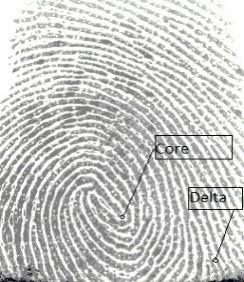(b)

## 1c.jpg(c)

Figure 1. Illustration of various fingerprint features (a) ridge and valley, (b) global features (core and delta), (c) local features (ridge ending and bifurcation)

## 2.png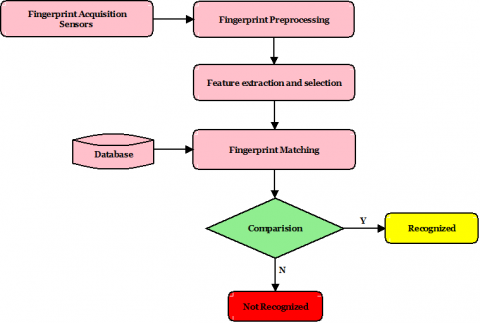Figure 2. Basic model (flowchart) of automated fingerprint recognition system

An automated fingerprint recognition system (AFRS) refers to the automated technique of verifying similarity between two human fingerprints, eliminating the need for human expertise. Each AFRS consists of two phases: (i) enrolment phase and (ii) recognition phase. In the registration phase, the person is directly registered by storing the person's fingerprint features in the database for future use. While in the recognition phase, the fingerprint features captured from a person are compared with all fingerprints whose features are stored in the database . Figure 2 shows the basic model for a fingerprint recognition system, with each phase broken down into the following sub-phases: (i) fingerprint acquisition, (ii) fingerprint image pre-processing, (iii) feature extraction, and (iv) database storage .

In the fingerprint capture step, fingerprint images are captured using sensors. Sometimes these fingerprint images are not suitable for processing due to less pressure, wetness, and dust on the sensor. Image pre-processing is the next and essential step to improve the quality of fingerprint images. The next step is feature extraction, which extracts features such as minutiae that are essential landmarks required for fingerprint recognition systems. It then stores these features in the fingerprint database. In the fingerprint recognition step, test fingerprint features are extracted and compared to the fingerprint database.

The performance of any fingerprint recognition system depends heavily on the adoption of efficient feature extraction and matching algorithms that reduce the probability of error in the recognition step. The quality of the fingerprint also plays a dominant role in the feature extraction step and depends on the skin condition, the person's age, and the sensor sensitivity. Therefore, it may be possible that the same finger creates different impressions at different instances due to the above factors. As a result, an AFRS system is required that can detect the similarities in all different fingerprint images of the same finger and match them to the same stored pattern . The Hopfield Neural Network (HNN) is known to perform the task in this way. It is a fully connected neural feedback network used to store patterns in a manner somewhat similar to the brain, and when a complete or partial pattern is given, it can recall complete patterns (see details in the study of Singh and Dixit ). But unfortunately, HNN suffers from limited storage capacity and inadequate recalling capability . In order to overcome these problems, this study uses a modified form of HNN known as Multi-Connections Hopfield neural network (MC-HNN) to develop an AFRS system. MC-HNN uses multiple connections among the neurons to store each pattern independently and recall the correct stored pattern using the Lyapunov energy function and Hamming distance . It caters to the need for storage capacity and inadequate recalling capability of HNN. Except this, by adding auto-associative memory in the form of MC-HNN, this system makes computation less complex and saves memory. Therefore, this study proposed a method to develop a fingerprint recognition system using MC-HNN that will work in a noisy environment.

The major contribution of this study is:

1. To propose a method for fingerprint recognition that utilizes MC-HNN, which improves accuracy and robustness in a noisy environment.
2. To pre-process the fingerprint images to make them suitable for the enrollment and matching operations.
3. To analyze the performance of the proposed method on fingerprint images of various datasets like FVC 2004, NIST4, and the Internal dataset.
4. To compare the performance of the proposed method in the presence of random noise in fingerprint patterns.
5. To compare the performance of the proposed method with some state of art methods.

This study contains six sections. Section 2 describes a literature review of recent previous work. Section 3 describes the MC-HNN in detail. Section 4 talks about the implementation of the proposed fingerprint recognition method, and Section 5 presents the experimental results and discussions. Finally, the conclusion is given in Section 6.

2. Literature Review

After exploring the above literature on automated fingerprint recognition technology, the authors gained a wide range of ideas on fingerprint identification and matching methods. Although much work has already been done in the field of fingerprint technology by various researchers, there is still room for improvement. The existing systems still suffer from accuracy, simplicity, storage capacity, noisy environment, and response time. Hence, this study develops a fingerprint recognition system with a multiconnection Hopfield neural network (MC-HNN) that works well in terms of accuracy and robustness to noise . Besides this, the proposed method adds MC-HNN as associative memory that makes use of complete fingerprints instead of extracting various types of features fingerprints that are complex to compute, store and match. Hence, this method makes fingerprint feature extraction and matching operations less complex and saves memory.

3. Multi-Connection Hopfield Neural Network (MC-HNN)

Associative memory (AM) stores patterns collectively in the form of weight matrix or memory and produces a complete pattern when triggered from incomplete versions of patterns . AMs can be either auto-associative or hetero-associative memory and implemented by artificial neural networks. Hopfield Neural Network (HNN) is a popularly used artificial neural network for auto-associative memory that mimics the functionality of the human brain [26, 27]. HNN is a recurrent network in which the output of each iteration is fed back as input in the next iteration using feedback connections and behaves like a nonlinear dynamic system that leads to generating multiple behavior patterns. One possible behavior pattern is the convergence of the network at a motionless point or fixed point. Considering this capability of the network, the same fixed point can be treated as input and output for such networks. This keeps the network in the same state. The network also shows oscillations or chaotic behavior. It has been shown that HNN can function as a stable system with multiple fixed points. The convergence of the network to a fixed point can be determined by the starting point chosen at the beginning of the iteration. In the case of the Hopfield neural network, these fixed points are called attractors, and a set of points attracted to a particular attractor during iteration is known as a basin of attraction. All points that are part of the basin of attraction are connected to an attractor. The major limitations of HNN are its limited storage capacity, tolerance to limited noise ratio, and cross-association . Therefore, a modified version of HNN called MC-HNN is used to overcome these limitations.

The architecture of MC-HNN is shown in Figure 3, where each neuron is connected to other neurons through multiple connections . The connection weight between ‘i’ and ‘j’ neurons for the kth connection is given by $w_{i j}^{k}\left(\right.$ with $w_{i j}^{k}=w_{j i}^{k}$ and $\left.w_{i i}^{k}=0\right)$. During the learning phase, each pattern is stored using the specific connection (for example, the kth pattern is stored in the network by using kth connection among all neurons and so on), whose result is an etalon array corresponding to a particular connection. Therefore, the weight matrix $W_{n \times n \times k}$  is determined by the collection of these etalon arrays (where n is the size of the patterns and k is the number of connections). The computation method and snapshot of weight matrix for MC-HNN are shown in Figure 4 (a) and 4(b) respectively.

In this architecture, the stability of states (i.e., global minima) is determined by computing the energy value of each pattern using the Lyapunov function and is given by Eq. (1):

$E(p)=-\frac{1}{2} \sum_{i=1}^{n} \sum_{j=1}^{n} s_{i}(p) s_{j}(p) w_{i j}^{p}$,

for $p=1,2, \ldots, P$ and $\theta=0$                  (1)

where, each S(p)=(s1(p), s2(p),.., si(p),..., sn(p)) is the pth stored pattern.

## 3.jpgFigure 3. A snapshot of MC-HNN architecture 

## 4.png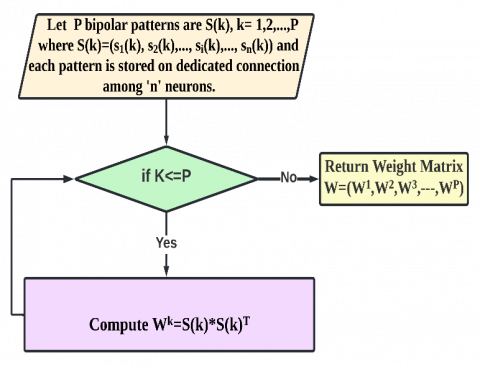(a)

## 4b.png(b)

Figure 4. (a) Computation of weight matrix, (b) Pictorial representation of weight matrix of MC-HNN

During the convergence phase, the recall of a complete pattern corresponding to a noisy version of the input pattern is quite challenging because each etalon array contains a stable state. This problem can be solved by computing the energy value of the input pattern corresponding to each etalon array using the Lyapunov energy function given in Eq. (2). The etalon arrays with computed minimum energy values are selected as attractors because they show the input pattern's affinity. In simple words, the input pattern can be treated as a point in the attraction basin corresponding to these selected etalon arrays.

$S E(p)=-\frac{1}{2} \sum_{i=1}^{n} \sum_{\substack{j=1 \\ j \neq i}}^{n} I_{i} I_{j} w_{i j}^{p}$,

for $p=1,2, \ldots, P$ and $\theta=0$                      (2)

After that, output vectors are computed corresponding to each selected etalon array using asynchronous updates. Eq. (2) and (4) presents the computation of output patterns using the input units i=1, 2,…, n in random order (asynchronous update units) and also computes the energy value corresponding to output patterns that lead to convergence of input pattern to output pattern at minimum energy value using Eq. (5).

$O_{n e t_{i}}(p)=I_{i}+\sum_{j} O_{j}(p) w_{i j}^{p} \text {, }$

for $\mathrm{p}=1,2, \ldots, \mathrm{P}$                (3)

O_{i}(p)=\left\{\begin{aligned} 1 & \text { if } O_{n e t_{i}}(p)>\theta \\ 0 & \text { if } O_{n e t}(p)==\theta \\-1 & \text { if } O_{n e t_{i}}(p)<\theta \end{aligned}\right\}                      (4)

$S E 1(p)=-\frac{1}{2} \sum_{i=1}^{n} \sum_{j=1}^{n} O_{i}(p) O_{j}(p) w_{i j}^{p}$,

for $p=1,2, \ldots, P$ and $\theta=0$                  (5)

To get the correct output, the affinity of the input pattern to attraction basin(s) of various motionless points can be determined by computing the minimum value of energy function of SE(p) (for p=1,2,…,P), i.e., min (SE (1), SE (2), SE (3), …, SE (P) ). In the second step, select the output pattern corresponding to the etalon array with the minimum energy function value. If more than one output pattern seems to be the candidate solution, then Hamming distance (HD) plays a vital role in determining the correct output pattern. HD is used to determine the number of bit differences in two binary vectors [27, 28]. A pattern with a smaller HD from the input test pattern gives the correct output pattern.

To ensure the output pattern's stability, we compared the energy value of the output pattern with the energy value of the stable point of the stored pattern. If the energy value of both is found to be the same, then recalled pattern is considered stable (or correct); otherwise, not.

In the subsequent section, the learning phase and convergence phase algorithm of MC-HNN is discussed in considerable detail.

3.1 Learning phase

During the learning phase, the weight matrix is computed, which is determined by computing etalon arrays corresponding to each pattern. Etalon arrays thus obtained are stored in the weight matrix. For these etalon arrays to act as attractors, the weight matrix is defined using the following algorithm.

3.2 Convergence phase

This phase is also known as the testing phase of an MC-HNN that is used to test the network with noisy or partial patterns for perfect recalling of stored patterns corresponding to noisy patterns.

This algorithm is also be summurized through flowgraph as shown in Figure 5.

Algorithm 1: Learning algorithm to store patterns in MC-HNN

1. Let store a set of bipolar patterns S(p), p=1…P where $S(p)=\left(s_{1}(p), s_{2}(p), \ldots, s_{i}(p), \ldots, s_{n}(p)\right)$ the etalon arrays corresponding to each pattern is defined as:

$W_{i j}^{p}=s_{i}(p) s_{j}(p) \quad$ for $i \neq j$                   (6)

where, the weights have no self-connections i.e., $w_{i j}^{p}=0$ for i=j.

1. The weight matrix is a collection of the etalon arrays given below:

$W=\left(W^{1}, W^{2}, W^{3} \ldots \ldots \ldots \ldots, W^{p}\right)$                  (7)

## 5.pngFigure 5. Flowchart of convergence phase

Algorithm 2: Recall of stored pattern

1. Initialize the weight matrix as per the Hebb rule for the bipolar pattern given in Eq. (7).
2. Calculate energy function value E(p) (for p=1,2,..., P) corresponding to each etalon arrays and associated patterns using Eq. (1).
3. For the input testing pattern, compute the energy function value SE(p) (for p=1, 2,..., P) corresponding to each etalon array and input pattern using Eq. (2).
4. Compute the output patterns O(p) (for p=1, 2,...,P) corresponding to the input pattern and each etalon array by the asynchronous update and using Eqns. (3) and (4).
5. Compute the energy function value SE1(p) (for p=1, 2,..., P) for output pattern corresponding to etalon array using Eq. (6).
6. To get the correct output, the affinity of the input pattern to attraction basin/s of various motionless points or attractors is identified by determining the minimum energy function value/s of SE (p) (for p=1, 2,...,P), i.e., min (SE (1), SE (2), SE (3),……… SE (P) ).
7. Select the output pattern/s corresponding to the minimum energy function value as given in step 6.
8. If more than one output pattern is the candidate solution, select the pattern with minimum Hamming distance from the input test pattern.
1. Compare the energy function values E(p) and SE1(p) to check the output pattern's stability; if both the values are found to be the same, then the pattern is correctly recalled, otherwise print an error message.
4. Proposed Fingerprint Recognition System

The framework of the proposed AFRS is illustrated in Figure 6. The system is divided into three major steps, namely pre-processing, recognition, and identification, wherein unknown fingerprints are used as input. In the pre-processing step, several operations are performed to improve the quality of fingerprint images. In the recognition step, MC-HNN AM is used to authenticate and identification of these fingerprint images. The proposed AFRS is further explained in subsequent sections.

4.1 Pre-processing of fingerprint images

The fingerprint images are used in this study are taken from the various datasets and not of perfect quality, thus requiring image enhancement techniques to reveal finer details from fingerprint images which may be uncovered due to improper impression or the poor resolution of the sensor. In this study, fingerprint images are pre-processed by Histogram Equalization (HE), Fast Fourier Transform (FFT), Binarization . A histogram is the graphical representation of the distribution of gray levels present in an image. HE is used to expanding the gray level distribution of an image that results in increased perceptional data. Figure 7 illustrates the results of HE on fingerprint images.

After histogram equalization, these fingerprint images are subjected to fast Fourier transformation to preserve the finer details. This method divides the fingerprint image into smaller square blocks of size 2k, where k ϵ Z and FFT of each block is computed according to Eq. (8).

$F(u, v)=\sum_{x=0}^{M-1} \sum_{y=0}^{N-1} f(x, y) \times e^{-j 2 \pi\left(\frac{u x}{M}+\frac{v y}{N}\right)}$

for, $u=0,1,2,3,31$ and $v=0,1,2,3, \ldots, 31$                        (8)

In order to improve a block by its dominant frequencies, the FFT of a block is multiplied by its magnitude (i.e., |F(u,v)|) by a set number of times (k). The value of k is experimentally determined and set to .35 here to calculate. To find the new enhanced block in the frequency domain, inverse FFT is performed according to Eq. (9).

$g(x, y)=F^{-1}\left(F(u, v) \times|F(u, v)|^{k}\right)$                  (9)

where, F-1(F(u,v)) is given below by the Eq. (10)

$f(x, y)=\sum_{x=0}^{M-1} \sum_{y=0}^{N-1} F(u, v) \times e^{j 2 \pi\left(\frac{u x}{M}+\frac{v y}{N}\right)}$

for, $x=0,1,2,3,31$ and $y=0,1,2,3, \ldots, 31$                          (10)

## 6.png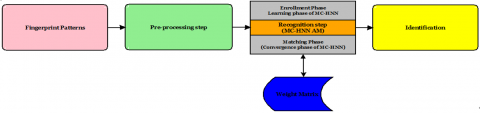Figure 6. Framework of proposed fingerprint recognition system

## 7.jpg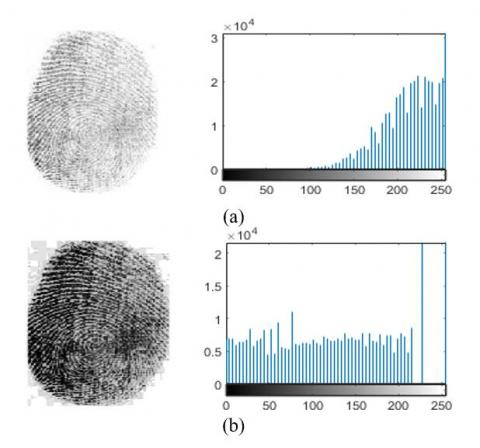Figure 7. Result of histogram equalization (a) original fingerprint image and corresponding histogram (b) histogram equalized image and corresponding histogram

## 8a.png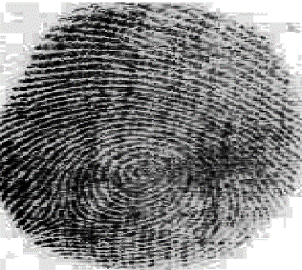(a)

## 8b.png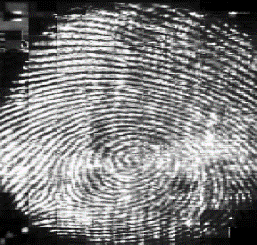(b)

Figure 8. Fingerprint enhancement using FFT (a) Histogram equalized fingerprint image (b) FFT enhanced fingerprint image

Figure 8 shows the FFT enhanced fingerprint in which falsely broken points on the ridges are connected, and false connections between the ridges are removed.

Binarization is a crucial step in fingerprint pre-processing because the true information that could be extracted from the fingerprint is binary, i.e., ridge and valleys. If grayscale fingerprint images are considered for further processing, then intensities of ridges may vary and create a problem to decide the presence of ridges. Therefore, binarization transforms a 256-level grayscale image into a 2-level image with the same information. This study used thresholding and Bradley techniques to convert modified grayscale images into binary images by assigning 0-value for ridges and 1-value for valleys. The Bradley technique works in the locally adaptive manner in which the entire image is divided into smaller blocks and based on the mean intensity of each block, binarization is performed . Hence, the advantage of using the Bradley technique is better binarization and faster execution time. Figure 9 shows the results of binarization step after global thresholding (Figure 9(a)) and Bradley techniques (Figure 9(b)).

## 9a.jpg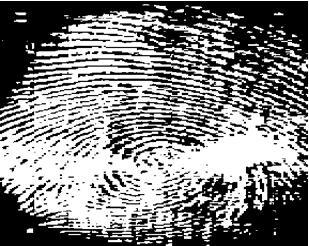(a)

## 9b.jpg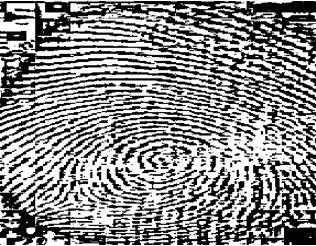(b)

Figure 9. Binary fingerprint images after (a) Thresholding technique (b) Bradley technique

## 10.png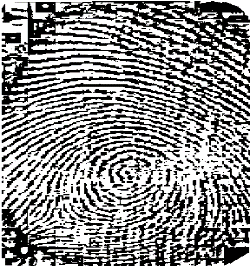Figure 10. ROI of fingerprint image

Now the speed of AFRS can be increased by clipping and cropping the fingerprint area containing the fingerprint feature (ROI) and only needing to process 50% of the finger features, not all. Figure 10 shows the cropped ROI of the fingerprint image. The cropped fingerprint images are resized into 30×30 pixels. It can also be noted here that the speed of AFRS increases without reducing the accuracy of the system. The fingerprint images obtained after cropping and resizing is converted into bipolar patterns, with each pixel having value +1 and -1. These bipolar patterns are converted into a bipolar vector of size 900×1. This conversion reduces the data to be processed and preserve image-related information.

4.2 Recognition of fingerprint images

Algorithm 3: Proposed fingerprint recognition system algorithm

1. Enrolment Phase
1. 1 Initialize the fingerprint dataset.
2. 2 Pre-process fingerprint images and convert them into bipolar patterns.
3. 3 Fingerprint patterns are processed by MC-HNN as per learning phase algorithms (Algorithm 1).
4. 4 Stored result in the form of weight matrix of MC-HNN.
2. Matching phase
1. 1 Select an unknown fingerprint image for recognition purposes.
2. 2 Pre-process an unknown fingerprint image and convert it into a bipolar pattern.
3. 3 Unknown fingerprint pattern is now processed as per convergence phase of MC-HNN (Algorithm 2)
4. 4 Compute the energy function of the output pattern.
5. 5 If the output pattern’s energy function value is the same as the energy value of the input stored pattern corresponding to the etalon array of the weight matrix, then fingerprint is identified otherwise not identified.

This step makes use of MC-HNN in two phases: (i) enrolment phase (ii) matching phase. In the enrolment phase, each fingerprint pattern obtained from pre-processing step is stored in MC-HNN in the form of a weight matrix and used for the next stage. Meanwhile, in the matching phase, an unknown input fingerprint pattern is presented to MC-HNN in order to recall a stored pattern corresponding to the input pattern, and recognition is performed based on that output pattern. The major advantage of using MC-HNN is the recalling of the complete pattern corresponding to noisy or incomplete input patterns. Hence, this system provides robustness in a noisy environment. The additive advantage of this AFRS is the scalability that is achieved by adding the additional pattern in the form of an etalon array to the weight matrix obtained during the enrolment phase. Figure 11 shows the working of the proposed AFRS in the form of two phases and described by Algorithm 3.

## 11.pngFigure 11. Enrolment and machining phases of proposed AFRS using MC-HNN

5. Experimental Results and Discussion

The Experimental results and discussion of the proposed AFRS are presented in this section. This study performed all experiments on a personal computer with Intel 7th Gen Core i7-7700, 4GB NVIDIA GTX 1050 Ti Graphics card, 64 GB RAM, Window 10(64-bit) operating system. The program of proposed AFRS is implemented on MATLAB (R2019a) using neural network and image processing toolboxes.

5.1 Datasets

The performance of our proposed AFRS is tested on three datasets, namely FVC 2004 , International NIST-4 , and the internal database. The detailed description of each dataset is discussed below.

1. FVC 2004 contains eight different fingerprint images of 80 fingers, so a total of 640 fingerprint images. However, this study used only 80 fingerprints of individuals. Fingerprint images of different sizes are arranged in DB1, DB2, DB3, DB4 of size 640×480, 328 × 364, 300×480, and 288 ×384, respectively; and each having a resolution of 500 dots per inch .
2. The internal dataset contains 2000 grayscale fingerprint images of size 300×400 of different individuals collected from college.
3. The International NIST4 database contains two fingerprint impressions of 2000 individuals, so a total of 4000 grayscale fingerprint images of size 512×512. This study used unique 2000 fingerprint images of each individual .

It is also noted here all datasets used fingerprint images are stored in .tiff (tag image file) format that is immune to any deterioration and loss and provides the highest quality format for commercial use. In addition, this format is more versatile and eliminates the need for any compression.

5.2 Evaluation criteria

The performance of the proposed method is evaluated on the following evaluation matrices:

1. False Rejection Rate (FRR): it is the measure of the likelihood that AFRS will wrongly reject access of authorized user and is defined as follows:

$F R R=\frac{\text { Total number of rejected persons }}{\text { total number of persons }}$

1. Total Success Rate (TSR): It is the likelihood that ARFS will correctly accept access of authorized users and defined as follows:

$T S R=\frac{\text { Total number of matched persons }}{\text { total number of persons }}$

5.3 Result and discussion

In this section, the performance of the proposed AFRS is analyzed and compared with some state of art methods. In order to evaluate the performance of the proposed AFRS, a total of three experiments are performed. In experiment 1, the performance of the proposed method is evaluated for all three given datasets by applying pre-processing step. Experiment 2 is carried out to prove the robustness of the proposed system under a noisy environment, and experiment 3 is performed to show the behavior of the system at different size fingerprint patterns and noise ratios.

Experiment 1: This experiment is performed to evaluate the performance of the proposed AFRS on all three datasets. The experiment shows that only 9 patterns out of 2000 and 12 patterns out of 2000 were not recognized by the system from the internal and NIST4 datasets, respectively, while all patterns were recognized from the FVC (2004). The detailed evaluation matrices for each dataset are given in Table 1.

It is observed from the table that the average accuracy of the proposed AFRS is 99.65% for all datasets. The binary techniques (Thresholding and Bradley) also play a significant role in the performance of the proposed system. By using the internal dataset, the threshold technique achieves an accuracy of 98.95% and fails to recognize 21 patterns out of 2000 patterns. This shows that the Bradley technique has higher accuracy compared to the threshold technique and is shown in Figure 12.

Experiment 2: This experiment is performed to show the robustness of the proposed system in a noisy environment. Here, the test fingerprint pattern is distorted by explicitly introducing random noise up to percentage of 0%, 10%, 20%, 30%, 40% and 50% by modifying 0, 90,180, 270, 350,450 bits respectively. The accuracy results for each dataset under various percentage noise are shown in Table 2.

It is observed from Table 2 that the average accuracy of the proposed system is satisfactory, and a minor degradation is seen as noise percentage in finger patterns increases. This is due to the presence of MC-HNN AM that retrieved complete pattern when triggered from the noisy pattern. Hence, the proposed AFRS performs well as compared to existing AFRSs in noisy environments. In the existing AFRSs, extraction of poor-quality fingerprint images is required to avoid errors in recognition and directly raise questions on the process of making low-quality images. The proposed AFRS performs better in poor-quality fingerprint images; therefore, the elimination of poor-quality images is not required, which saves the processing time of the system.

Table 1. Performance analysis of proposed system

 Dataset Used Number of samples Recognized Patterns Unrecognized Patterns TSR FRR Recognition Rate (%) FVC 2004 80 80 0 1 0 100 NIST 4 2000 1991 9 0.9955 0.0045 99.55 Internal 2000 1988 12 0.9940 0.0060 99.40

## 12.pngFigure 12. Accuracy comparison between binary techniques

Table 2. Accuracy (%) of the proposed system at various noise percentages in fingerprint patterns

 Datasets Accuracy on various noise percentages in fingerprint patterns 0% 10% 20% 30% 40% 50% FVC 2004 100 100 100 98.75 95.00 91.25 NIST 4 99.55 99.55 99.40 99.15 98.80 98.70 Internal 99.40 99.40 99.10 98.85 98.55 98.25

Table 3. Average accuracy (in percentage) of the proposed system with varying size fingerprint patterns in the noisy environment

 Noise Percentage Size of fingerprint patterns (in pixels) 30×30 40×40 50×50 0% 99.65 100 100 10% 99.65 100 100 20% 99.50 100 100 30% 98.92 99.60 100 40% 97.45 98.98 100 50% 96.07 98.30 99.80

Table 4. Comparative analysis of our proposed system with other ANN-based states of the art methods

 S. No. Authors Dataset used Accuracy (%) 1. Alam et al.  Internal dataset of 128 fingerprint images. 68.75% 2. Mahmood  Internal dataset of 560 fingerprint images 89.00% 3. Qader et al.  FVC 2002 dataset 92% 4. Kouamo and Tangha  BDAL dataset of 500 fingerprint images 94.70% 5. Azzoubi and Ibrahim  FVC 2000,FVC 2002, FVC 2004 98.00% 6. Tamrakar and Gupta  FVC2002 83.00% 7. Almajmaie et al.  NIST 4, FVC 2004, Internal Dataset 99.56% 8. Chatterjee et al.  FVC 2002 95.00% 9. The Proposed Method NIST 4, FVC 2004, Internal Dataset 99.65%

## 13.png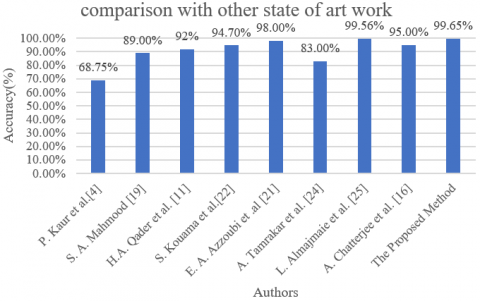Figure 13. Average accuracy-based comparison of the proposed system with some state of art methods used in literature

Experiment 3: This experiment is conducted to show the behavior of proposed AFRS in noisy environments by varying the size of fingerprint images. In this experiment, fingerprint images of different sizes viz 30×30, 40×40, and 50×50 pixels are used. The average accuracy results for each dataset at 0 to 50% noise is depicted in Table 3.

It is observed from Table 3 that the average accuracy of the proposed system increases as fingerprint pattern size increases. It is due to the MC-HNN AM that easily selects the correct attraction basin for large size noisy test fingerprint patterns that result in greater average accuracy.

In order to show the effectiveness of the proposed AFRS, its performance is compared with other state of art methods. The accuracy  obtained by these methods is compared with the proposed method and is given in Table 4 and Figure 13.

The results given in Table 4 show that the proposed method is superior to other state-of-the-art methods in terms of accuracy and achieved 99.65% accuracy. Therefore, the proposed system performs better for fingerprint recognition as compared to other state-of-the-art methods.

6. Conclusion

This study presented an innovative idea for an automatic fingerprint recognition system using MC-HNN to identify individuals. The application of MC-HNN as associative memory in the proposed system makes it more accurate and robust in noisy environments. Moreover, MC-HNN works on complete patterns instead of various levels of features extracted from fingerprint images. Hence, it reduces the complexity of the fingerprint recognition process by eliminating the feature extraction step and also saves the memory that is required to store fingerprint features. The proposed method achieved promising results with 99.65% accuracy, which is higher than the other existing methods. Experimental results showed that the proposed method also works well in noisy environments. The performance comparison with the state-of-the-art methods indicates the effectiveness of the proposed system.

The results demonstrate that the proposed system can easily be extended into a commercial fingerprint biometric system. Hence, the future scope of this study is to develop a highly efficient and accurate fingerprint biometric system that shows low complexity and requires lesser space.

References

 Jain, A.K., Bolle, R., Pankanti, S. (2009). Biometrics Personal Identification in Networked Society. Springer Science & Business Media.

 Jain, A.K., Pankanti, S. (2001). Automated Fingerprint Identification and Imaging Systems. In: Lee, H.C., Gaensslen, R.E. (eds.) Advances in Fingerprint Technology, 2nd edition, 275-326.

 Maltoni, D., Maio, D., Jain, A.K., Prabhakar, S. (2009). Handbook of Fingerprint Recognition. Springer Science & Business Media.

 Alam, A.N., Ahsan, M., Based, M.A., Haider, J., Kowalski, M. (2021). An intelligent system for automatic fingerprint identification using feature fusion by Gabor filter and deep learning. Computers & Electrical Engineering, 95: 107387. https://doi.org/10.1016/j.compeleceng.2021.107387

 Yadav, J.K.P.S., Jaffery, Z.A., Singh, L. (2020). A short review on machine learning techniques used for fingerprint. Journal of Critical Review, 7(13): 2768-2773. https://doi.org/10.31838/jcr.07.13.419

 Egawa, S., Awad, A.I., Baba, K. (2012). Evaluation of Acceleration Algorithm for Biometric Identification. In: Benlamri, R. (ed.) NDT 2012, Part II. CCIS, 294: 231-242.

 Kaur, P., Kaur, J. (2013). Fingerprint recognition using genetic algorithm and neural network. International Journal of Computational Engineering Research, 3(11): 41-46.

 Singh, M.P., Dixit, R.S. (2013). Optimization of stochastic networks using simulated annealing for the storage and recalling of compressed images using SOM. Engineering Applications of Artificial Intelligence, 26(10): 2383-2396. https://doi.org/10.1016/j.engappai.2013.07.003

 Yadav, J.K.P.S., Jaffery, Z.A., Singh, L. (2017). Comparative analysis of recurrent networks for pattern storage and recalling of static images. International Journal of Computer Applications, 170(10): 0975-8887.

 Yadav, J.K.P.S., Jaffery, Z.A., Singh, L. (2022). Optimization of Hopfield neural network for improved pattern recall and storage using Lyapunov energy function and hamming distance: MC-HNN. International Journal of Fuzzy System Applications (IJFSA), 11(2).

 Qader, H.A., Ramli, A.R., Al-Haddad, S.A. (2007). Fingerprint recognition using Zernike moments. The International Arab Journal of Information Technology, 4(4): 372-375.

 Gu, J., Zhou, J., Yang, C. (2006). Fingerprint recognition by combining global structure and local cues. IEEE Transactions on Image Processing, 15(7): 1952-1964. https://doi.org/10.1109/TIP.2006.873443

 Park, U., Pankanti, S., Jain, A.K. (2008). Fingerprint verification using SIFT features. SPIE Defense and Security Symposium, Orlando, Florida, 69440-69440. https://doi.org/10.1117/12.778804

 Jain, A., Chen, Y., Demirkus, M. (2007). Pores and ridges: High-resolution fingerprint matching using level 3 features. IEEE Transactions on Pattern Analysis and Machine Intelligence, 29(1): 15-27. https://doi.org/10.1109/TPAMI.2007.250596

 Alijla, B.O., Saad, M., Issawi, S.F. (2017). Neural network-based minutiae extraction for fingerprint verification system. In Proceedings of 8th International Conference on Information Technology (ICIT), pp. 435-441. https://doi.org/10.1109/ICITECH.2017.8080039

 Chatterjee, A., Mandal, S., Rahaman, G.A., Arif, A.S.M. (2010). Fingerprint identification and verification system by minutiae extraction using artificial neural network. JCIT, 1(1): 12-16.

 Wang, R., Han, C., Wu, Y., Guo, T. (2016). A novel fingerprint classification method based on deep learning. In Proceeding of 23rd International Conference on Pattern Recognition (ICPR), pp. 926-931.

 Jeon, W.S., Rhee, S.Y. (2017). Fingerprint pattern classification using convolution neural network. International Journal of Fuzzy Logic and Intelligent Systems, 17(3): 170-176.

 Mahmood, S.A. (2014). Fingerprint recognition system using support vector machine and neural network. International Journal of Computer Science Engineering and Information Technology Research (IJCSEITR), 4(1): 103-110.

 Patil (Waghjale), S.R., Suralkar, S.R. (2013). Neural network based fingerprint classification. International Journal of Science and Research (IJSR), 2(1): 58-62.

 Azzoubi, E.A., Ibrahim, R.B. (2015). An enhancement algorithm using Gabor filter for fingerprint recognition. Journal of Theoretical and Applied Information Technology, 74(3): 355-363.

 Kouamo, S., Tangha, C. (2016). Fingerprint recognition with artificial neural networks: Application to E-learning. Journal of Intelligent Learning Systems and Applications, 8(2): 39-49. https://doi.org/10.4236/jilsa.2016.82004

 Appati, J.K., Nartey, P.K., Owusu, E., Denwar, I.W. (2021). Implementation of a transform-minutiae fusion-based model for fingerprint recognition. International Journal of Mathematics and Mathematical Sciences, 5545488: 1-12. https://doi.org/10.1155/2021/5545488

 Tamrakar, A., Gupta, N. (2020). Low resolution fingerprint image verification using CNN filter and LSTM classifier. International Journal of Recent Technology and Engineering (IJRTE), 8(5): 3546-3549.

 Almajmaie, L., Ucan, O.N., Bayat, O. (2019). Fingerprint recognition system based on modified multi-connect architecture (MMCA). Cognitive Systems Research, 58: 107-113. https://doi.org/10.1016/j.cogsys.2019.05.004

 Hopfield, J.J. (1982). Neural networks and physical systems with emergent collective computational abilities. Proceedings of the National Academy of Sciences, 79(8): 2554-2558. https://doi.org/10.1073/PNAS.79.8.2554

 Hamming, R.W. (1950). Error detecting and error correcting codes. The Bell System Technical Journal, 29(2): 147-160. https://doi.org/10.1002/j.1538-7305.1950.tb00463.x

 Kareem, E.A., Kareem, A.L., Ali, W.A.H., Jantan, A. (2012). MCA: A developed associative memory using multi-connect architecture. Intelligent Automation and Soft Computing, 18(3): 291-308.

 Perichappan, K.A.P., Sasubilli, S. (2017). Accurate fingerprint enhancement and identification using minutiae extraction. Journal of Computer and Communications, 5(14): 28-38. https://doi.org/10.4236/jcc.2017.514003

 Bradley, D., Roth, G. (2007). Adaptive thresholding using the integral image. Journal of Graphics Tools, 12(2): 13-21. https://doi.org/10.1080/2151237X.2007.10129236

 FVC2004 web site: http://bias.csr.unibo.it/fvc2004, accessed on 24 Oct. 2021.

 NIST-4 website: https://www.nist.gov/srd/nist-specialdatabase-4, accessed on 10 Nov. 2021.

 Singh, L., Alam, A., Kumar, K.V., Kumar, D., Kumar, P., Jaffery, Z.A. (2021). Design of thermal imaging based health condition monitoring and early fault detection technique for porcelain insulators using machine learning. Environmental Technology & Innovation, 24: 102000.